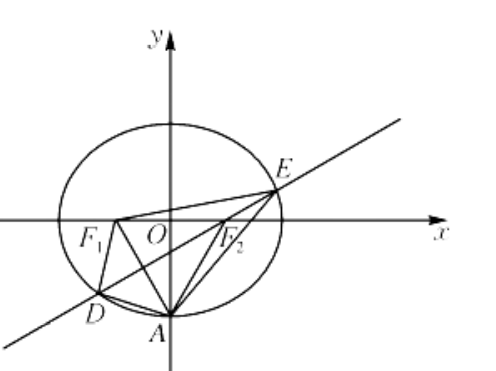【答案】 $\frac{52}{3}$

【解析】 因为 $C$ 的离心率 $e=\frac{c}{a}=\frac{1}{2}$, 所以 $a=2 c, b^2=a^2-c^2=3 c^2$, 所以 $C$ 的䇞程为 $\frac{x^2}{4 c^2}+\frac{y^2}{3 c^2}=1$, 即 $3 x^2+4 y^2-12 c^2=0$. 在 $\triangle A F_1 F_2$ 中, $\left|A F_1\right|=\left|A F_2\right|=a=2 c,\left|F_1 F_2\right|=2 c$, 所以 $\triangle A F_1 F_2$ 为正三角形. 过 $F_2$ 且垂直于 $A F_1$ 的直线与 $C$ 交于 $D, E$ 两点, 所以 $D E$ 为线段 $A F_1$ 的 垂直平分线, 直线 $D E$ 的斜率为 $\frac{\sqrt{3}}{3}$, 所以直线 $D E$ 的方程为 $y=$ $\frac{\sqrt{3}}{3}(x-c)$. 设 $D\left(x_1, y_1\right), E\left(x_2, y_2\right)$, 由 $\left\{\begin{array}{l}3 x^2+4 y^2-12 c^2=0, \\ y=\frac{\sqrt{3}}{3}(x-c)\end{array}\right.$ 得 $13 x^2$

$-8 c x-32 c^2=0$, 所以 $x_1+x_2=\frac{8 c}{13}, x_1 x_2=-\frac{32 c^2}{13}$, 所以 $|D E|=\sqrt{1+k^2}\left|x_1-x_2\right|=\sqrt{1+\frac{1}{3}}$. $\sqrt{\left(\frac{8 c}{13}\right)^2-4 \cdot\left(-\frac{32 c^2}{13}\right)}=\frac{48}{13} c=8$. 解得 $c=\frac{13}{6}$. 所以 $a=2 c=\frac{13}{3}$. 因为 $D E$ 为线段 $A F_1$ 的垂直平分线, 所以 $|A D|=\left|D F_1\right|,|A E|=\left|E F_1\right|$, 所以 $\triangle A D E$ 的周长为 $|A D|+|A E|+|D E|=\left|D F_1\right|+\left|D F_2\right|+$
$$\left|E F_1\right|+\left|E F_2\right|=2 a+2 a=4 a=\frac{52}{3} .$$